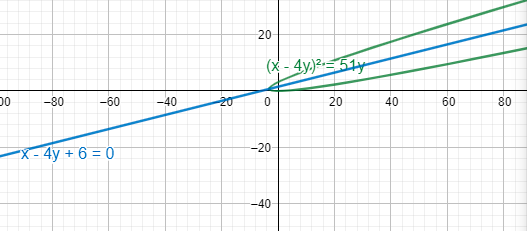Courses
Courses for Kids
Free study material
Free LIVE classes
MoreLIVE
Join Vedantu’s FREE Mastercalss

# To trace the required parabola:${{(x-4y)}^{2}}=51y$Verified
360.3k+ views
Hint: To solve this problem, one needs to be aware about the generalised equation of a parabola. This equation is given by ${{\left( \dfrac{px+qy+r}{\sqrt{{{p}^{2}}+{{q}^{2}}}} \right)}^{2}}=4a\left( \dfrac{lx+my+n}{{{l}^{2}}+{{m}^{2}}} \right)$. Here, a is the focus of the parabola. Further; p, q, r, l, m and n are the constants which will one comes across as the equation is solved. We will compare this equation with one given in the problem to trace the parabola to get the value of these constants.

To solve this problem, one must be aware about the general equation of parabola in detail. Generally, one is aware about the equation ${{y}^{2}}=4ax$ where there is no xy term (Here, a is the length of the focus of the parabola). However, in this problem as we expand the terms of the question, there will be a xy term, thus we make use of a different generalised equation. Thus, first to begin with, we expand the terms, we get,
${{(x-4y)}^{2}}=51y$
${{x}^{2}}+16{{y}^{2}}-8xy=51y$
Now, we try to compare with the below equation –
${{\left( \dfrac{px+qy+r}{\sqrt{{{p}^{2}}+{{q}^{2}}}} \right)}^{2}}=4a\left( \dfrac{lx+my+n}{{{l}^{2}}+{{m}^{2}}} \right)$-- (1)
Now, to start solving, we use a small trick, we introduce a variable (in this case k). To explain,
${{(x-4y+k)}^{2}}=51y+2kx-8ky+{{k}^{2}}$
(Thus, to make the equation to compare to (1), we do this manipulation. Also, since, we have added k in the square term on LHS, we have to subsequently add the terms on RHS for equality to hold true.)
${{(x-4y+k)}^{2}}=2kx+(51-8k)y+{{k}^{2}}$ -- (A)
Thus, we have two line equations –
x-4y+k=0 -- (2)
2kx+(51-8k)y+${{k}^{2}}$=0 -- (3)
These lines are perpendicular to each other since these are the equations of the axis and line perpendicular to the axis passing through the vertices of the parabola.
Let slope of (2) be m and slope of (3) be n. Thus,
m=$\dfrac{1}{4}$
n=$\left( \dfrac{-2k}{51-8k} \right)$
We have mn=-1 (condition of perpendicular lines)
Thus,
$\Rightarrow$ $\dfrac{1}{4}\times \left( \dfrac{-2k}{51-8k} \right)$=-1
Solving, we get,
\begin{align} & -2k=-4(51-8k) \\ & -2k=-204+32k \\ & 34k=204 \\ & k=6 \\ \end{align}
We put this value in (A), we get,
\begin{align} & {{(x-4y+6)}^{2}}=12x+(51-48)y+36 \\ & {{(x-4y+6)}^{2}}=12x+3y+36 \\ & {{(x-4y+6)}^{2}}=3(4x+y+12) \\ & {{(x-4y+6)}^{2}}=4\times \dfrac{3}{4}\times (4x+y+12) \\ & {{\left( \dfrac{x-4y+6}{\sqrt{17}} \right)}^{2}}=4\times \dfrac{3}{4}\times \dfrac{1}{\sqrt{17}}\times \dfrac{(4x+y+12)}{\sqrt{17}} \\ \end{align}
Thus, we can finally compare this to (1)
${{\left( \dfrac{px+qy+r}{\sqrt{{{p}^{2}}+{{q}^{2}}}} \right)}^{2}}=4a\left( \dfrac{lx+my+n}{{{l}^{2}}+{{m}^{2}}} \right)$
We can find, the constants value by comparing,
p=1, q=-4, r=6, a=$\dfrac{3}{4\sqrt{17}}$, l=4, m=1, n=12
To get the vertices, we have to solve the equations
x-4y+6=0 and 4x+y+12=0
Solving, we get,
x=$\dfrac{-54}{17},y=\dfrac{12}{17}$
Thus, the required parabola will have the above vertices with axis as x-4y+6=0. This will look as –Note: While solving questions related to parabola apart from the familiar equation ${{y}^{2}}=4ax$, we should also be aware of the general equation of the parabola given by ${{\left( \dfrac{px+qy+r}{\sqrt{{{p}^{2}}+{{q}^{2}}}} \right)}^{2}}=4a\left( \dfrac{lx+my+n}{{{l}^{2}}+{{m}^{2}}} \right)$. This equation is especially useful when parabola involves xy terms in its equation.
Last updated date: 24th Sep 2023
Total views: 360.3k
Views today: 9.60k